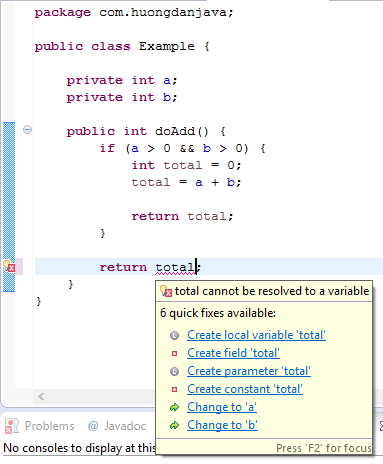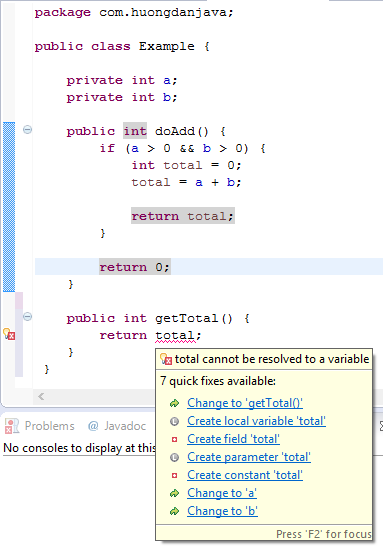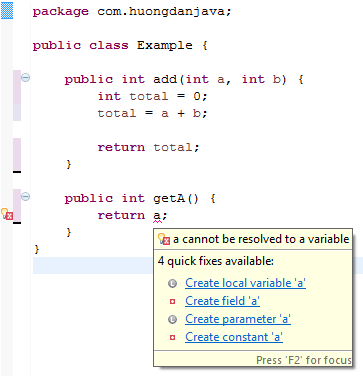# The scope of variables in Java – Part 1

In Java, any variable can have the following scopes:

• The variable is inside any method.
• As a parameter of any method.
• The variable is inside an object (including fields, attributes, and variables that are not static variables).
• The static variable of a class.

In this tutorial, we will explore the first two scopes of a variable in Java 😀

### The variable is inside a method

When a variable is in a method, it can be in an if else statement, in a for loop, while, do while, or in a switch statement. We often use this variable to store a temporary calculation result and they are the smallest scope variables.

For example:

In the example above, the total variable is defined in the doAdd() method and in an if statement.

We cannot access variables defined in a method from outside of that method, or if it is defined within an if else statement, loop, or switch statement, the variable cannot be accessed from outside of those statements.

For example:In this example, the total variable cannot be accessed from outside the if statement.

The following example shows how the total variable cannot be accessed from outside the method.In a nutshell, a variable is defined in a method, the ability to access it depends on its location in that method.

• If it is in an if else, a loop, a switch statement, or a block ({}), then it can only be accessed within these statements.
• If it is outside of all the above statements, this variable can be accessed anywhere within that method.

Variables defined in one method are not accessible from other methods.

### Variable is a parameter of a method

A variable is defined as a parameter of the method, the access to that variable is contained in the method.

For example:

Here variables a and b are defined as parameters of the add() method. They are only accessible from within this method. If we try to access them outside of the add() method, we will have a compile error.Variables defined as a parameter of a method are accessed anywhere in a method, within an if else statement, loop, or switch statement. So you see these variables have a greater reach than the variables defined in a method.

In the next tutorial, let’s take a look at the remaining two scopes of variables in Java.